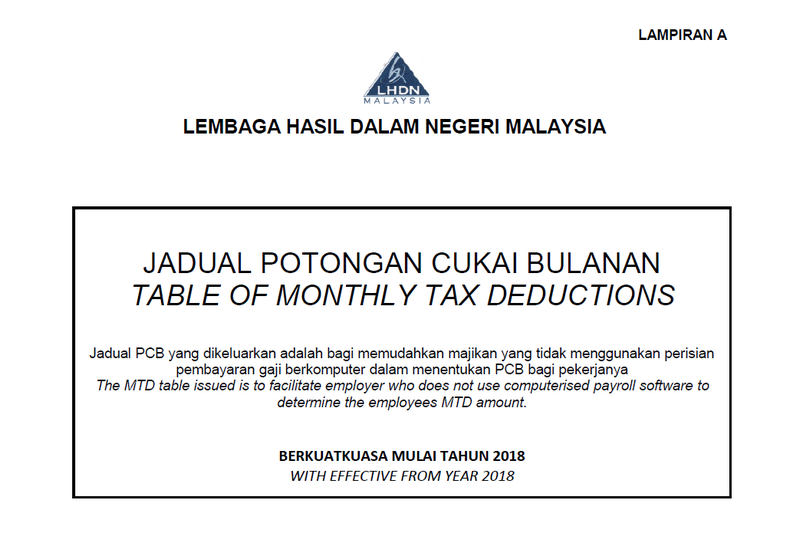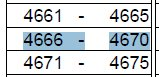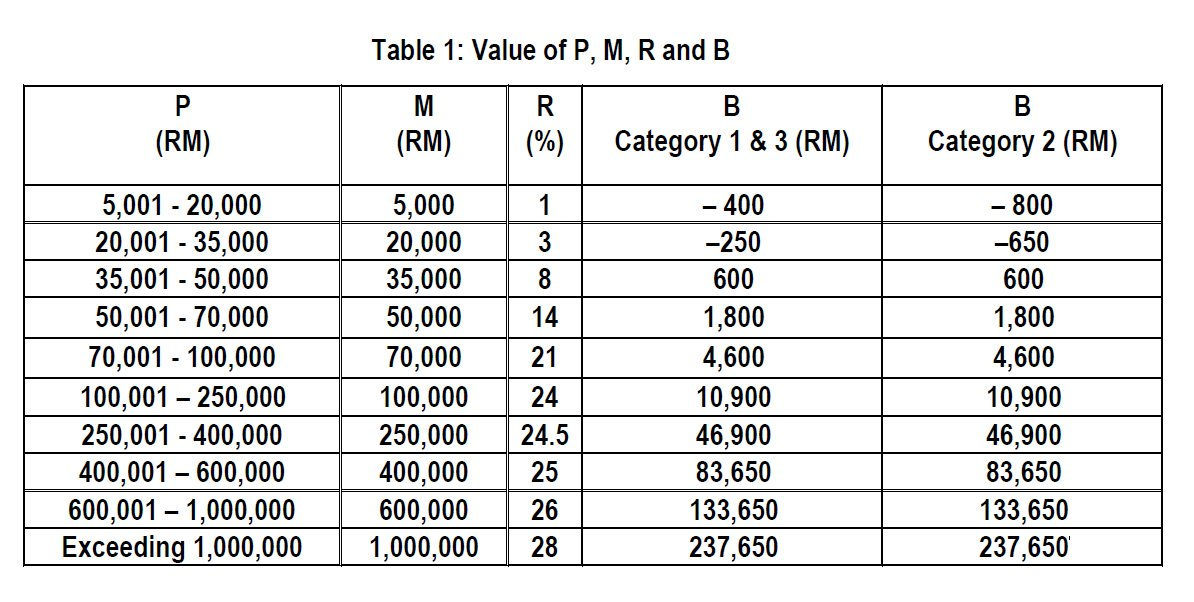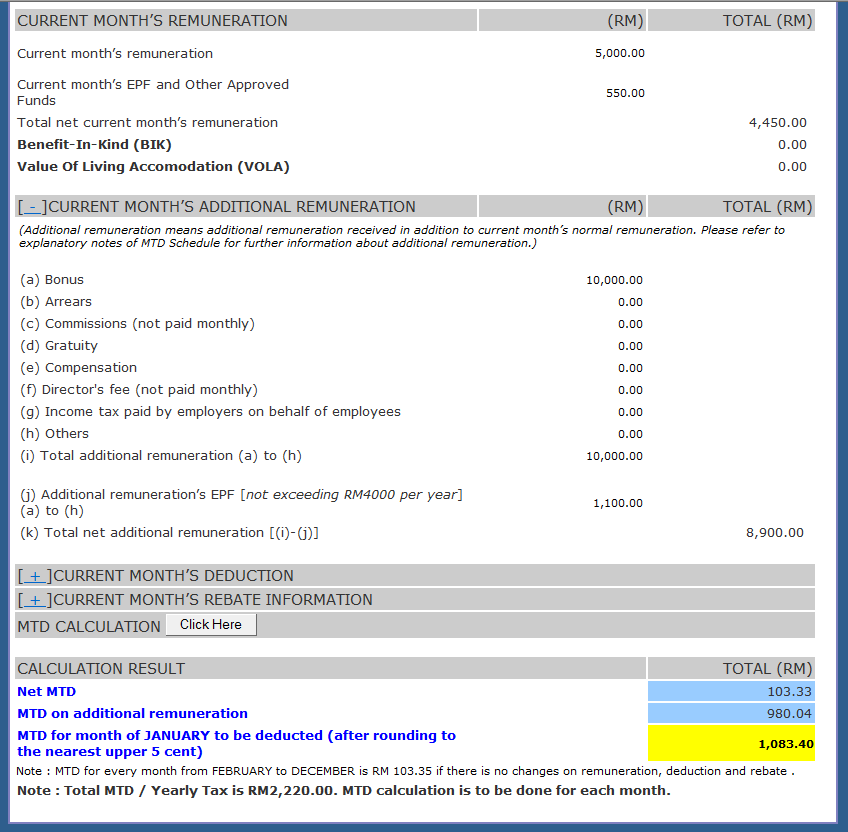# 3 Ways to do PCB calculation for Bonus without a Payroll Software – Updated for 2019

The Bonus PCB calculation was one of the most difficult part of writing the Actpay payroll software. We recommend using a software as everything is fast and easy as all the calculations are automatic, but there are many who still need do this manually.

This detailed article shows you step by step how to do the PCB calculation without using a software or the Online PCB calculator.

We have a lot of traffic for this post as manual bonus calculation is quite complicated. We have designed an Excel sheet to simplify the task of doing the Bonus PCB calculation. It makes the calculations much easier. Please read Manual PCB calculation Variation 1 first before using it.Download our free Trial version of Actpay which can be run for 40 days. Enough to do Bonus calculations .

Go Straight to Examples

Bonus PCB Calculation Issues

In our our research on PCB calculation using the MTD schedule, we found conflicting explanations online on how to calculate Bonus PCB. Over the years, the rules for calculating additional income has also changed. Most of the examples available are actually out of date.

Taking into account how the MTD Schedule is derived and the difference results between manual and computerized income tax calculations we have come up with 3 methods for calculating Bonus PCB and have provided a detailed explanation on the reason for the differing results.

Bonus PCB Calculation Explanation

Calculating PCB for bonus and additional income is quite difficult because of the complex calculations required. We haven’t yet found a simple example and explanation of this process online or offline. Below we will attempt to give a simple and step by step explanation that hopefully everyone is able to understand.

This calculation method for bonus applies to all additional income including adhoc commission or other income. Below we outline 3 ways calculate the PCB for bonus without using a computer software. All the methods shown below will give valid results, although the answer will differ slightly for each method.

The basic principle of PCB calculation is to extrapolate the monthly wage over 12 months to arrive at the best possible estimate of the the annual wage.

Both the computerized and manual calculations are geared towards a best guess of what the total wage will be at the end of the year.

Calculating PCB using the MTD Schedule provided by LHDN is based on the principal of taking each months wages in isolation. Therefore accumulated (YTD) wages and PCB do not come into play. In computerized PCB calculation, the actual Year to date figures are used to come at a more accurate computation of PCB.

Please note that the PCB schedule for 2018 is used for 2019. There are no changes to the tax calculations for 2019. The major change is the annual EPF amount eligible for deduction has been reduced from RM6,000 to RM4,000.## Manual PCB calculation Variation 1

This is the example is based on the most common example of PCB calculation for bonus found online. It is similar to the example found on the LHDN examples for 2008 bonus calculations, found at : http://www.hasil.gov.my/goindex.php?kump=5&skum=3&posi=1&unit=5100&sequ=7

For these examples, we have made some changes to make it easier to follow.

Employee details :

• Employee Married, Spouse is working with 2 children below 18
• Monthly salary of RM4,000
• Bonus of RM8,000

CATEGORY 3 (KA2)

Edit
BASIC SALARY 5,000.00
EPF = 550.00
Maximum allowed EPF deduction per month for 2019 is RM333
(2019 rules : Max EPF deduction per annum RM4000 / 12)
(333.00)
Taxable Income 4,667.00PCB 4,666 to 4,670 from table (KA2)

103.60
Bonus 10,000.00
EPF 1,100.00
Maximum allowed EPF deduction per month for 2019 is RM333
0.00
Taxable Bonus Income (10,000 – 0) 10,000.00
Monthly estimated salary with bonus
Basic + (Taxable Bonus)/12
4,667.00 + (10,000 / 12) 5,500.67
PCB for 4,496 to 5,500  from table (KA2) 185.00
Tax difference with and without bonus
Total PCB – Basic PCB (185.00 – 103.60) 81.40
PCB for Bonus = Extra PCB x 12 (81.40 x 12) 976.80
Total PCB for month (976.80+103.60) 1080.40

## Manual PCB calculation Variation 2 Using Formula

Step 1 – PCB for Basic Pay from schedule

Taxable Amount for current month = RM5,000–RM333.33 = RM4,667.00

PCB amount from Table  = RM103.60

Total PCB for current year = [RM103.60 x 12 month] = RM1,243.20

Step 2 – Use formula to calculate P

P = [(Y – K) x 12] + [Σ(Yt1 – Kt1)] + (Yt – Kt) – (D + S + QC)

Y = Basic Income and K = EPF deducted for basic income.

Yt1 and Kt1 are not applicable as accumulated (YTD) values are not used in manual calculations.

Yt = additional income and Kt = EPF deducted for additional income.

Y = 5,000

K = 333.33 (5,000 / 12) – Limit per annum for EPF deduction is 4,000. It used to be 6,000 in previous years )

Yt = 10,000

Kt = 0, since maximum EPF of 333.33 already deducted.

D = personal deduction = 9,000

S = spouse deduction = 0 because spouse is working, else it is RM4,000 this year.

Q = No. of children, C = deduction per child = 2,000.

P = [(Y – K) x 12] + [Σ(Yt1 – Kt1)] + (Yt – Kt) – (D + S + QC)

= [(RM5,000–RM333.33) x 12] + [RM0.00 – RM0.00] +[RM10,000 – RM0]

– [RM9,000 + RM0.00 + RM4,000]

= RM56,000.04 + RM10+10000,000 – RM13,000

= RM53,000.04

(D = personal deduction 9000, S = 0, spouse, C = No. of Children = 2 x 2000)

Step 3 – Calculate Total Tax for Basic Pay + BonusTotal tax for a year = [(P – M) R + B]

= [(RM53,000.04 – RM50,000) x 14% + RM1,800]

= RM3,000.04 x 14% + RM1,800

= RM420.00 + RM1,800

= RM2,220.00

P, M, R and B are derived from the Income tax table, based on P = (50,001 – 70,000)

Step 4 – PCB for Bonus ( Additional Remuneration )

Annual (PCB with bonus – PCB of basic)

Bonus PCB = RM2,2220.00 – RM1,243.20 = RM 976.80

Step 5 – Total PCB for current month

=  RM103.60 + RM 976.80

= RM1,080.40

## Using Online PCB Income Tax Calculator

There are 2 options in using the online PCB calculator:

If you are using MTD schedule for your monthly PCB calculations, use “PREVIOUSLY NOT EMPLOYED IN CURRENT YEAR”. There are 2 reasons for this.

• You do not have to enter Y-T-D information
• This will simulate the MTD Schedule values, because it does not take into account Y-T-D information.

Basic  : 5,000, EPF = 550 ( You can insert the actual EPF as long as the total EPF is below 4,000. The online calculator will automatically calculate the real values)

Bonus : 10,000, EPF =  1,100 ( You can insert the actual EPF as long as the total EPF is below 4,000. The online calculator will automatically calculate the real values)

Using the Online PCB Calculator the total value obtained for month = 1083.40

The MTD of bonus (additional income) = 980.04 => 980.00

Basic PCB = RM103.60, Bonus PCB = RM 976.80 and Total PCB = RM1,080.40

There is a slight variation of only RM3.00 and perfectly acceptable. The difference is due to rounding for values in the MTD Schedule versus the exact calculations use in the Online PCB calculator.Discrepancy between MTD Schedule and PCB Online Calulator

Results from MTD Schedule :

MTD for 4,667.00 = 103.60*

MTD for 5,000.00 = 130.00**

Results from PCB Online Income Tax Calculator :

MTD for 4667.00 = 103.40*

MTD for 5,000.00 = 130.00**

* There is a discrepancy between the 2 values because the MTD schedule round the value between 4666 to 4670 instead of the actual value of 4667.00

** For round numbers the results will normally be the same. In some cases though because the Online PCB calculator rounds up to nearest 0.05, while the MTD schedule rounds to the nearest 0.05, the value from the online calculator can be RM0.05 higher.

As can be seen the values obtained using the Online PCB Calculator with the amount rounded to the nearest RM5, the values obtained are the same as the MTD schedule with a variance of 0.05 in some cases.If this is still too difficult, you can download our free Trial version of Actpay which can be run for 40 days. Enough to do Bonus calculations for January and February 2019.

Conclusion

In conclusion, it has to be noted that there are multiple ways to calculate PCB and some of the methods can be quite tedious. Considering the work involved and the high chance of errors in the calculation, it is recommended that the calculations be done very carefully. To avoid errors it is recommended that a payroll software be used.

The most common mistake in bonus calculations is the not including the basic salary and monthly allowances while calculating bonus. The bonus PCB is the PCB on the amount additional to the monthly gross income.

Although we have tried to make the example as simple as possible, it may still be quite difficult to understand. If you have any questions, please post a comment here or ask a question under “Q&A” and we will try our best to answer.

## The following article explains the differences between paying Bonus separately and paying bonus together with the basic pay.

The following article explains how bonus calculations are done in Actpay